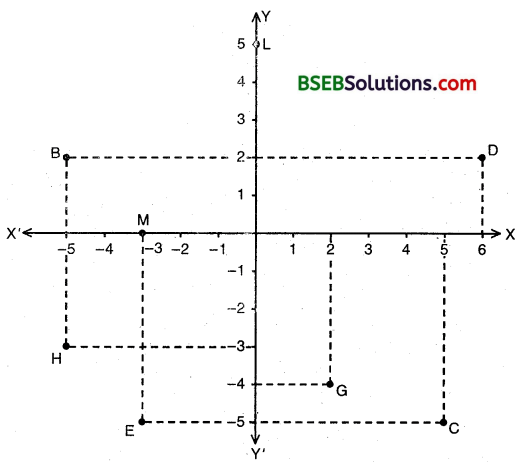# Bihar Board Class 9th Maths Solutions Chapter 3 Coordinate Geometry Ex 3.2

Bihar Board Class 9th Maths Solutions Chapter 3 Coordinate Geometry Ex 3.2 Textbook Questions and Answers.

## BSEB Bihar Board Class 9th Maths Solutions Chapter 3 Coordinate Geometry Ex 3.2Question 1.
Write the answer of each of the following questions :

1. What is the name of horizontal and the vertical lines drawn to determine the position of any point in the Cartesian plane?
2. What is the name of each part of the plane formed by these two lines?
3. Write the name of the point where these two lines intersect.

Solution:

1. The name of horizontal and vertical lines drawn to divide the plane into four parts is rectangular axes/coordinate axes.
2. The name of each part of the plane divided by axes is quadrant.
3. The axes intersects at the origin.Question 2.
See figure, and write the following :

1. The coordinates of B.
2. The coordinates of C.
3. The point identified by the coordinates (- 3, – 5).
4. The point identified by the coordinates (2, – 4).
5. The abscissa of the point D.
6. The ordinate of the point H.
7. The coordinates of point L.
8. The coordinates of the point M.Solution:
Clearly, from the figure :

1. The coordinates of B are (- 5, 2).
2. The coordinates of C are (5, – 5).
3. The coordinates (- 3, – 5) are identified by the point E
4. The coordinates (2, – 4) are identified by the point G
5. The abscissa of the point D is 6.
6. The ordinate of the point H is – 3.
7. The coordinates of the point L are (0, 5)
8. The coordinates of the point M are (- 3, 0).